Ground State Entanglement in 1 Dimensional TranslationallyInvariant Quantum

• Slides: 57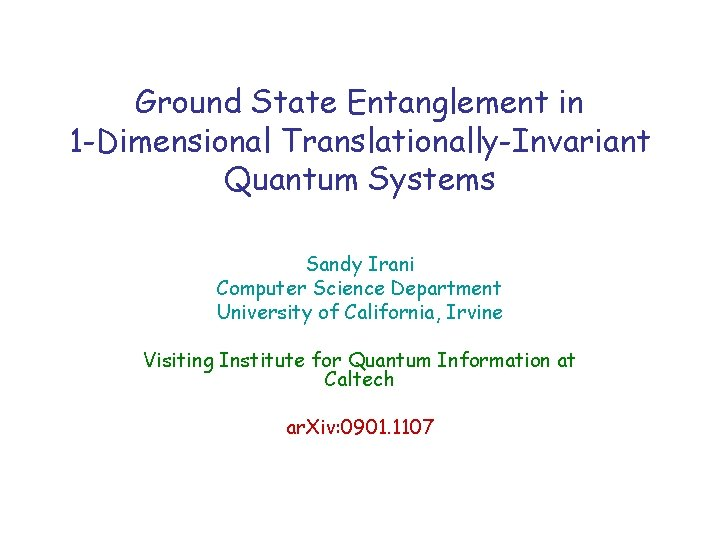Ground State Entanglement in 1 -Dimensional Translationally-Invariant Quantum Systems Sandy Irani Computer Science Department University of California, Irvine Visiting Institute for Quantum Information at Caltech ar. Xiv: 0901. 1107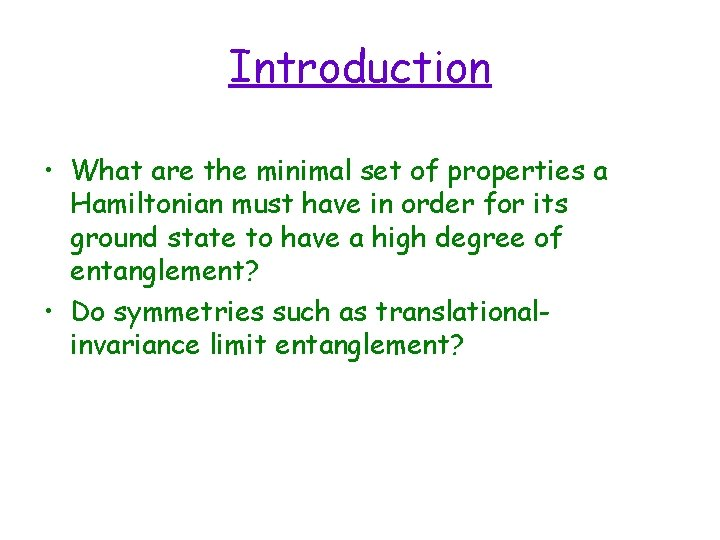Introduction • What are the minimal set of properties a Hamiltonian must have in order for its ground state to have a high degree of entanglement? • Do symmetries such as translationalinvariance limit entanglement?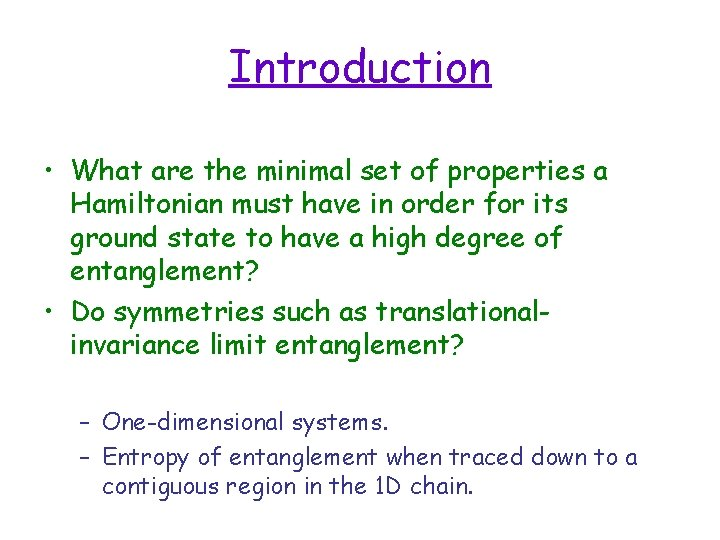Introduction • What are the minimal set of properties a Hamiltonian must have in order for its ground state to have a high degree of entanglement? • Do symmetries such as translationalinvariance limit entanglement? – One-dimensional systems. – Entropy of entanglement when traced down to a contiguous region in the 1 D chain.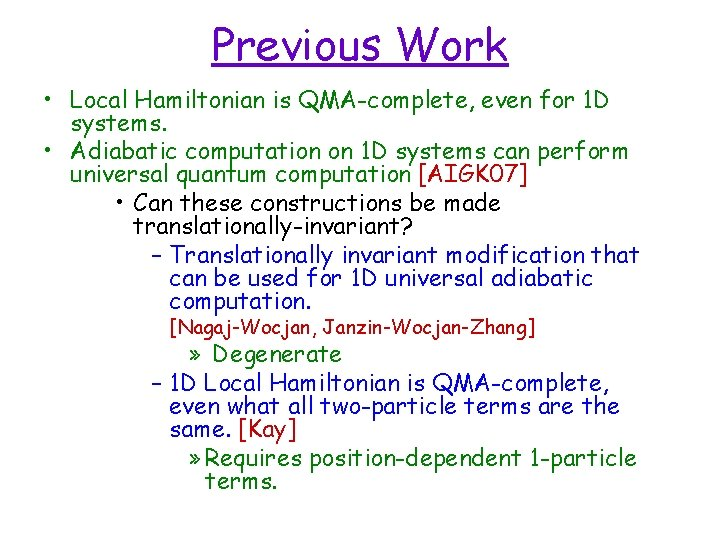Previous Work • Local Hamiltonian is QMA-complete, even for 1 D systems. • Adiabatic computation on 1 D systems can perform universal quantum computation [AIGK 07] • Can these constructions be made translationally-invariant? – Translationally invariant modification that can be used for 1 D universal adiabatic computation. [Nagaj-Wocjan, Janzin-Wocjan-Zhang] » Degenerate – 1 D Local Hamiltonian is QMA-complete, even what all two-particle terms are the same. [Kay] » Requires position-dependent 1 -particle terms.Translation-Invariance • Can we make the 1 D construction translationally-invariant with a Hamiltonian which has a non-degenerate ground state? • If the system is described by a single Hamiltonian term applied to all pairs of particles (with bounded precision), how do we encode a circuit? – Show high entanglement in the ground state. – For 1 D systems, previously known bounds, ground state entanglment entropy scales logarithmically with the region size.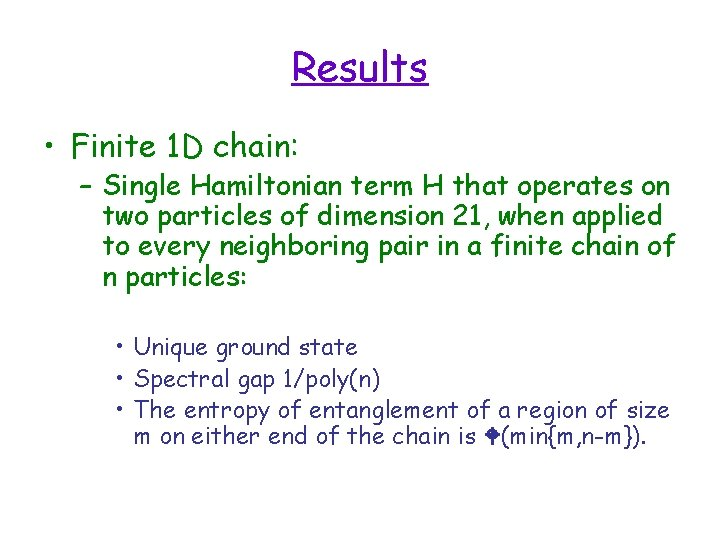Results • Finite 1 D chain: – Single Hamiltonian term H that operates on two particles of dimension 21, when applied to every neighboring pair in a finite chain of n particles: • Unique ground state • Spectral gap 1/poly(n) • The entropy of entanglement of a region of size m on either end of the chain is W(min{m, n-m}).Results • Cycles and the Infinite Chain: – Family of translationally-invariant Hamiltonians {Hn} for a cycle of nt 21 -dimensional particles • Spectral gap is 1/poly(n) • For any state in the ground space of Hn, and any m, there exist regions of size of m whose entanglement entropy is W (min{m, n}). – Entanglement bounds for a constant fraction of regions of size m. – Bounds hold in the limit as t tends towards infinity.1 D Area Law • Upper bound on the entanglement entropy for any ground state of a 1 D Hamiltonian H, independent of region size but exponentially dependent on 1/D, where D is the spectral gap of H. [Hastings 07] • Gottesman and Hastings: is the dependence on 1/D tight? – Family of 1 D Hamiltonians with unique ground state with regions whose entanglement entropy is W(poly(1/D)). – Previously, best known such lower bound was W(log(1/D)) • ar. Xiv: 0901. 1108 • Construction present here gives a similar result.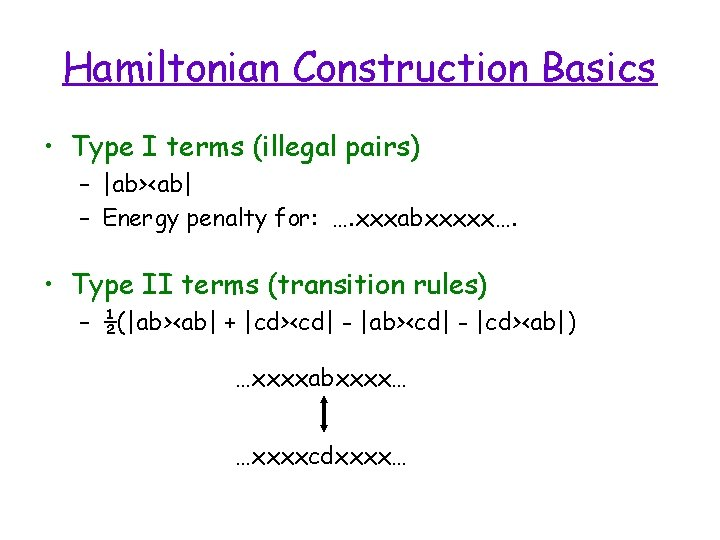Hamiltonian Construction Basics • Type I terms (illegal pairs) – |ab><ab| – Energy penalty for: …. xxxabxxxxx…. • Type II terms (transition rules) – ½(|ab><ab| + |cd><cd| - |ab><cd| - |cd><ab|) …xxxxabxxxx… …xxxxcdxxxx…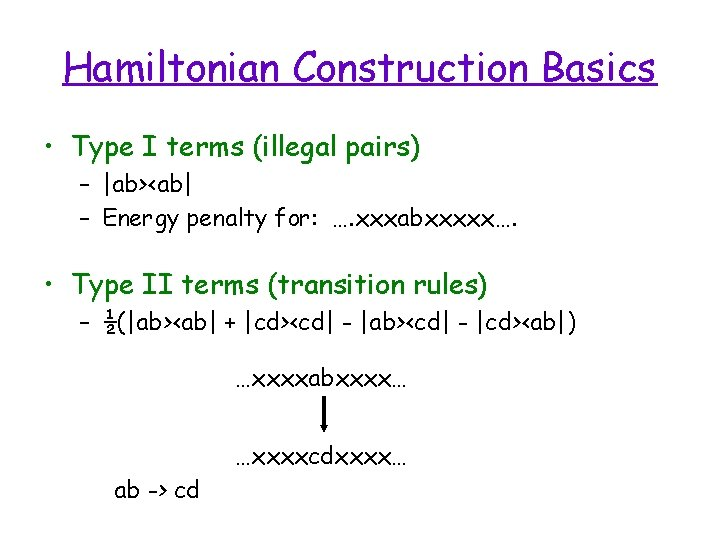Hamiltonian Construction Basics • Type I terms (illegal pairs) – |ab><ab| – Energy penalty for: …. xxxabxxxxx…. • Type II terms (transition rules) – ½(|ab><ab| + |cd><cd| - |ab><cd| - |cd><ab|) …xxxxabxxxx… …xxxxcdxxxx… ab -> cd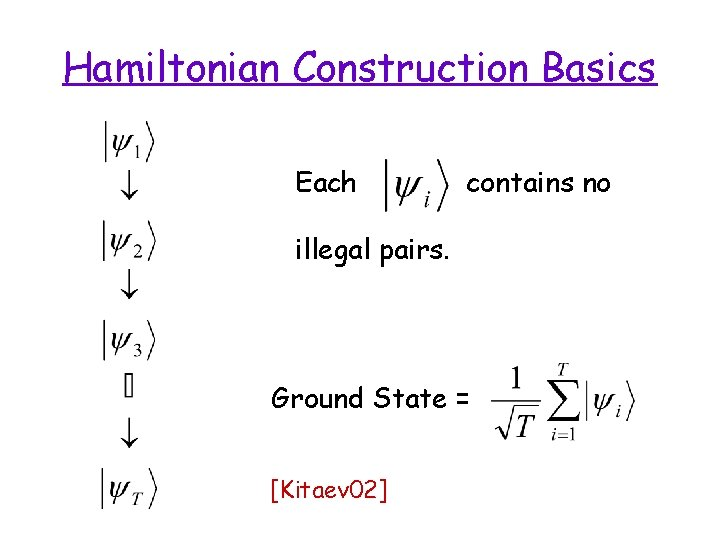Hamiltonian Construction Basics Each contains no illegal pairs. Ground State = [Kitaev 02]One Dimensional Hamiltonians • Two types of states: – Control states: h g f i I H – Passive States: W w E e U u < > • Transition rules apply to control state and a state to the right or left. – May move control state to the left or right. – For example: w f --> f W [AGIK 07]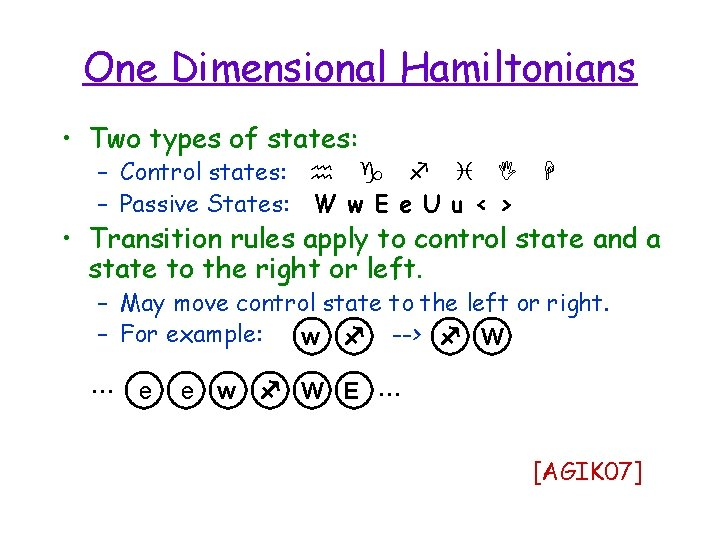One Dimensional Hamiltonians • Two types of states: – Control states: h g f i I H – Passive States: W w E e U u < > • Transition rules apply to control state and a state to the right or left. – May move control state to the left or right. – For example: w f --> f W … e e w f W E … [AGIK 07]One Dimensional Hamiltonians • Two types of states: – Control states: h g f i I H – Passive States: W w E e U u < > • Transition rules apply to control state and a state to the right or left. – May move control state to the left or right. – For example: w f --> f W … e e w f W E … [AGIK 07]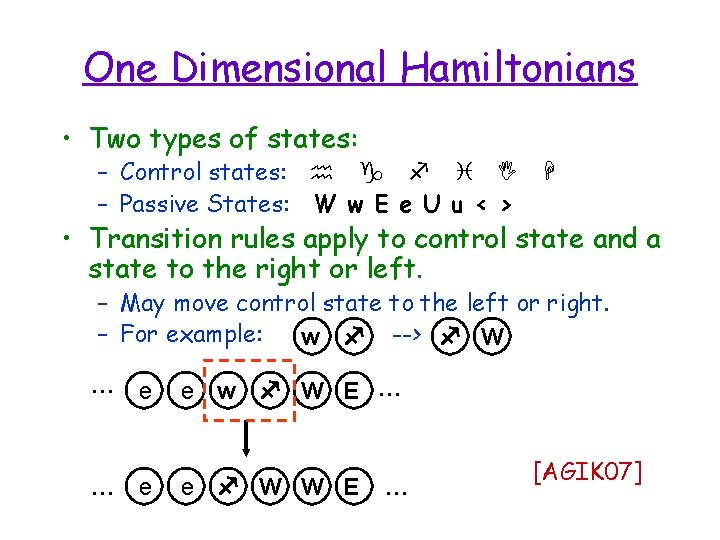One Dimensional Hamiltonians • Two types of states: – Control states: h g f i I H – Passive States: W w E e U u < > • Transition rules apply to control state and a state to the right or left. – May move control state to the left or right. – For example: w f --> f W … … e e e w f W E … e f W W E … [AGIK 07]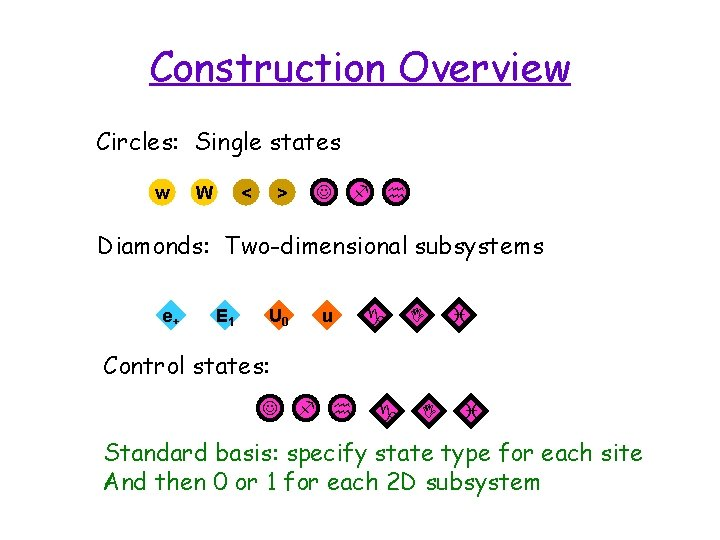Construction Overview Circles: Single states w W < > J f h Diamonds: Two-dimensional subsystems e+ E 1 U 0 u g I i Control states: J f h g I i Standard basis: specify state type for each site And then 0 or 1 for each 2 D subsystem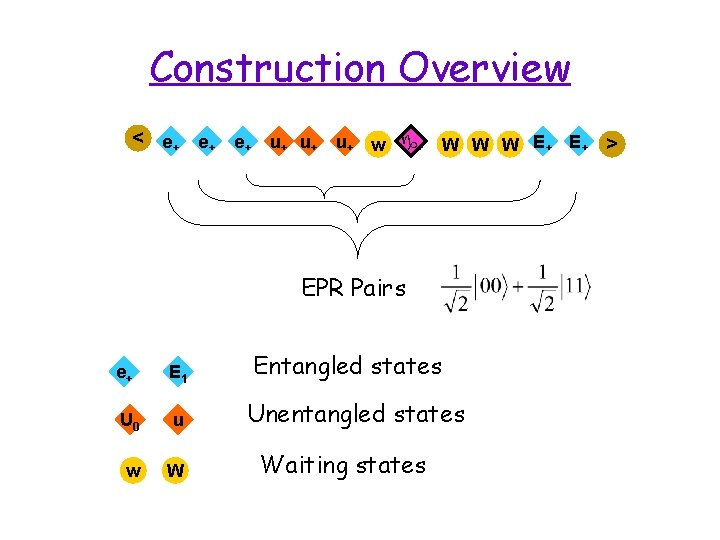Construction Overview < e+ e+ e+ u + u + w g+ W W W E + > EPR Pairs e+ E 1 U 0 u w W Entangled states Unentangled states Waiting statesTarget Ground State < e+ h U+ U+ W W E + >Target Ground State < e+ h U+ U+ W W E + >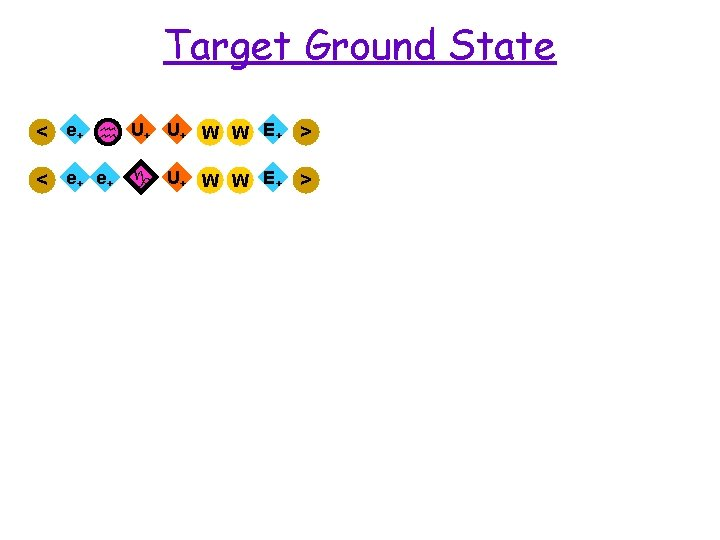Target Ground State < e+ h U+ U+ W W E + > < e+ e+ g U+ W W E + >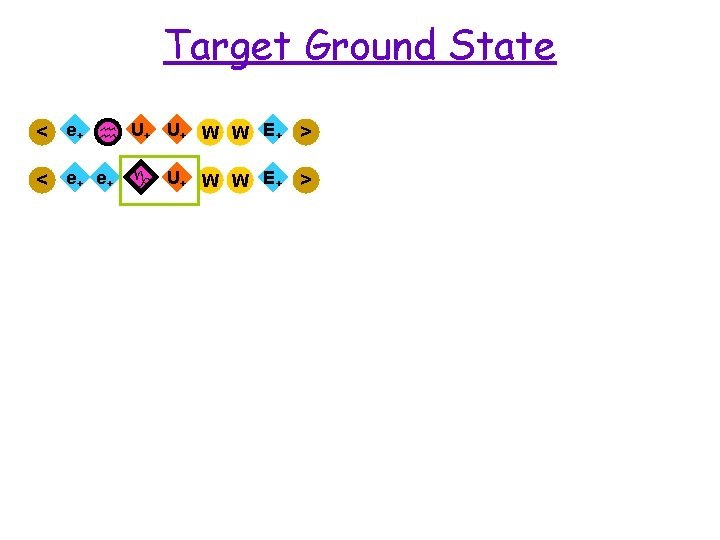Target Ground State < e+ h U+ U+ W W E + > < e+ e+ g U+ W W E + >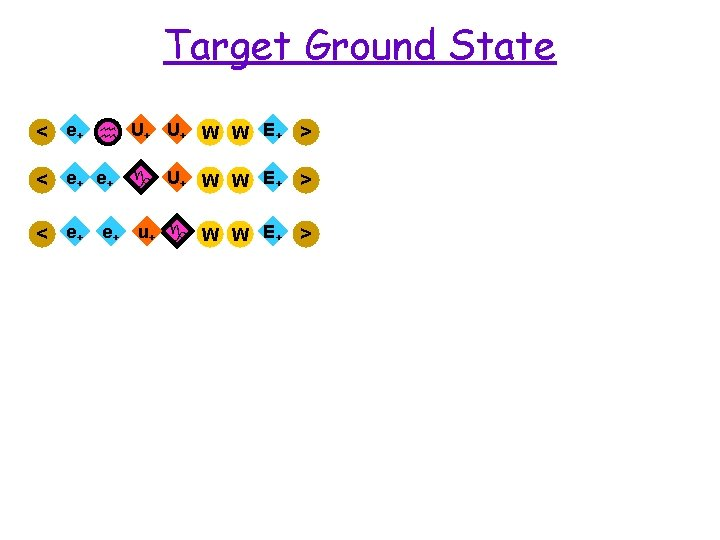Target Ground State < e+ h U+ U+ W W E + > < e+ e+ g U+ W W E + > < e+ e+ u + g W W E + >Target Ground State < e+ h U+ U+ W W E + > < e+ e+ g U+ W W E + > < e+ e+ u + g W W E + > < e+ e+ u + w g W E + >Target Ground State < e+ h U+ U+ W W E + > < e+ e+ g U+ W W E + > < e+ e+ u + g W W E + > < e+ e+ u + w g W E + > < e+ e+ u + w w g E+ >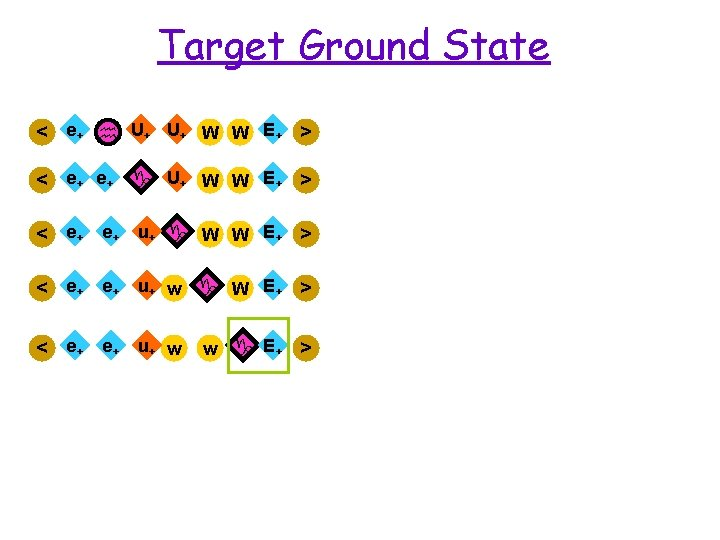Target Ground State < e+ h U+ U+ W W E + > < e+ e+ g U+ W W E + > < e+ e+ u + g W W E + > < e+ e+ u + w g W E + > < e+ e+ u + w w g E+ >Target Ground State < e+ h U+ U+ W W E + > < e+ e+ g U+ W W E + > < e+ e+ u + g W W E + > < e+ e+ u + w g W E + > < e+ e+ u + w w g E+ > < e+ e+ u + w w i E+ >Target Ground State < e+ h U+ U+ W W E + > < e+ e+ g U+ W W E + > < e+ e+ u + g W W E + > < e+ e+ u + w g W E + > < e+ e+ u + w w g E+ > < e+ e+ u + w w < e+ e+ u + w f E+ E+ > i E+ >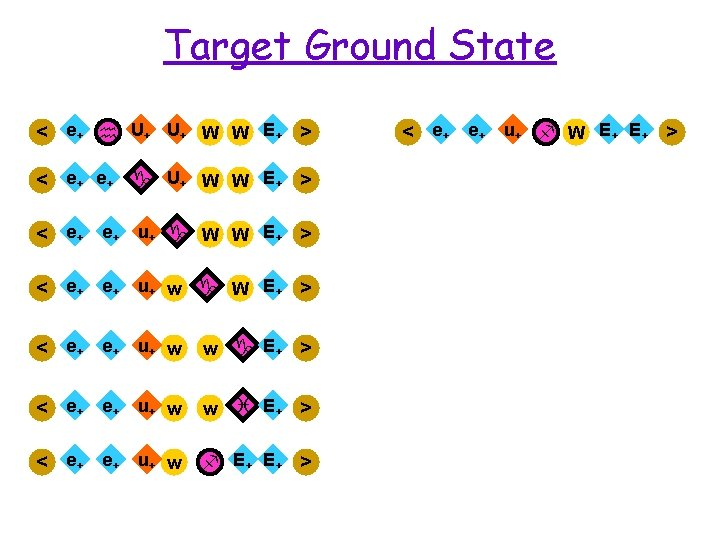Target Ground State < e+ h U+ U+ W W E + > < e+ e+ g U+ W W E + > < e+ e+ u + g W W E + > < e+ e+ u + w g W E + > < e+ e+ u + w w g E+ > < e+ e+ u + w w < e+ e+ u + w f E+ E+ > i E+ > < e+ e+ u + f W E + >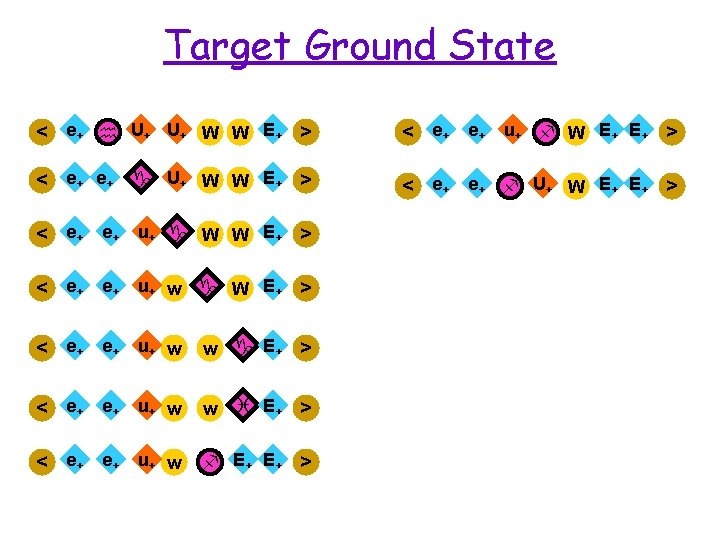Target Ground State < e+ h U+ U+ W W E + > < e+ e+ u + f W E + > < e+ e+ g U+ W W E + > < e+ e+ f U+ W E + > < e+ e+ u + g W W E + > < e+ e+ u + w g W E + > < e+ e+ u + w w g E+ > < e+ e+ u + w w < e+ e+ u + w f E+ E+ > i E+ >Target Ground State < e+ h U+ U+ W W E + > < e+ e+ u + f W E + > < e+ e+ g U+ W W E + > < e+ e+ f U+ W E + > < e+ e+ u + g W W E + > < e+ e+ u + w g W E + > < e+ e+ u + w w g E+ > < e+ e+ u + w w < e+ e+ u + w f E+ E+ > i E+ >Target Ground State < e+ h U+ U+ W W E + > < e+ e+ u + f W E + > < e+ e+ g U+ W W E + > < e+ e+ f U+ W E + > < e+ e+ u + g W W E + > < e+ e+ u + w g W E + > < e+ e+ u + w w g E+ > < e+ e+ u + w w < e+ e+ u + w f E+ E+ > i E+ > < e+ e+ h U+ W E + >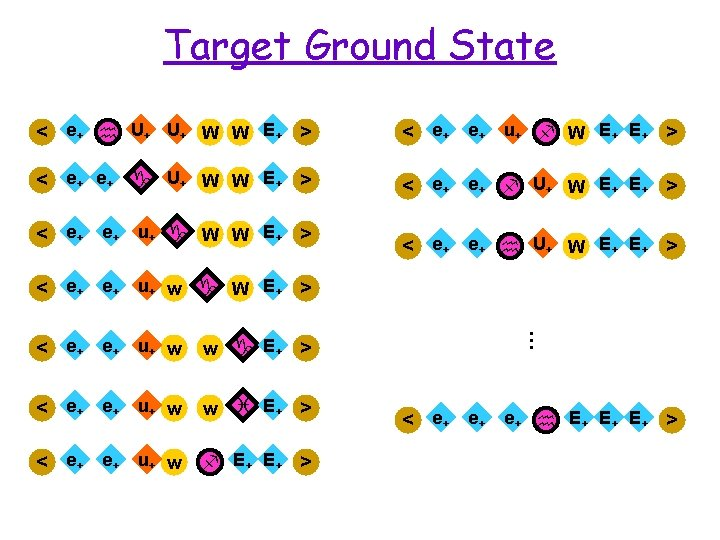Target Ground State < e+ h U+ U+ W W E + > < e+ e+ u + f W E + > < e+ e+ g U+ W W E + > < e+ e+ f U+ W E + > < e+ e+ u + g W W E + > < e+ e+ h U+ W E + > < e+ e+ u + w w g E+ > < e+ e+ u + w w < e+ e+ u + w f E+ E+ > i E+ > … < e+ e+ u + w g W E + > < e+ e+ e+ h E + E + >First Round < J U+ U+ U+ W W W > < e+ I U+ U+ W W W > < e+ u + I U+ W W W > < e+ u + u+ I W W W > < e+ u + u+ w I W W > < e+ u + u+ w w I W > w w I w w i > > Special control states for the first round force the left end and the right end to agree that it is the first round. Will be used to check that state is symmetric about center.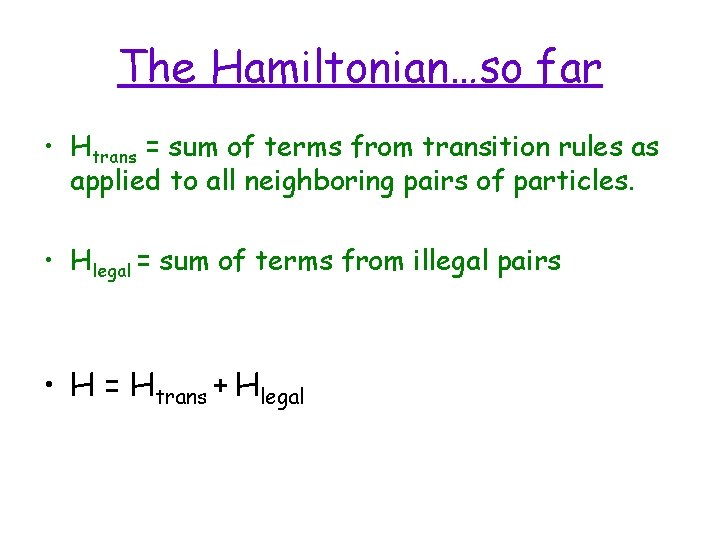The Hamiltonian…so far • Htrans = sum of terms from transition rules as applied to all neighboring pairs of particles. • Hlegal = sum of terms from illegal pairs • H = Htrans + HlegalWell-Formed States • A state in the standard basis is well-formed if it is of the form: – (<+e)(e)*(w+u)*(g+I+f)(W+U)*(E)*(>+e) – (<+e)(e)*(h+J)(W+U)*(E)*(>+e) – (<+e)(e)*(w+u)*(i)(E)*(>+e) • …or any substring of these forms • Can be checked by local checksWell-Formed States • Can not enforce by local checks that a legal state has a control state. – E. g. , eeee……eee must be a legal state. • If the state is bracketed ( a < on left end a > on the right end), then a legal state must be exactly on of the following three forms: – (<+e)(e)*(w+u)*(g+I+f)(W+U)*(E)*(>+e) – (<+e)(e)*(h+J)(W+U)*(E)*(>+e) – (<+e)(e)*(w+u)*(i)(E)*(>+e)Properties of Well-Formed States • Htrans + Hlegal is closed over the subspace spanned by well-formed states. – (All additional terms will be diagonal in standard basis). • For each well-formed state, at most one transition rule applies in the forward direction and at most one transition rule applies in the reverse direction.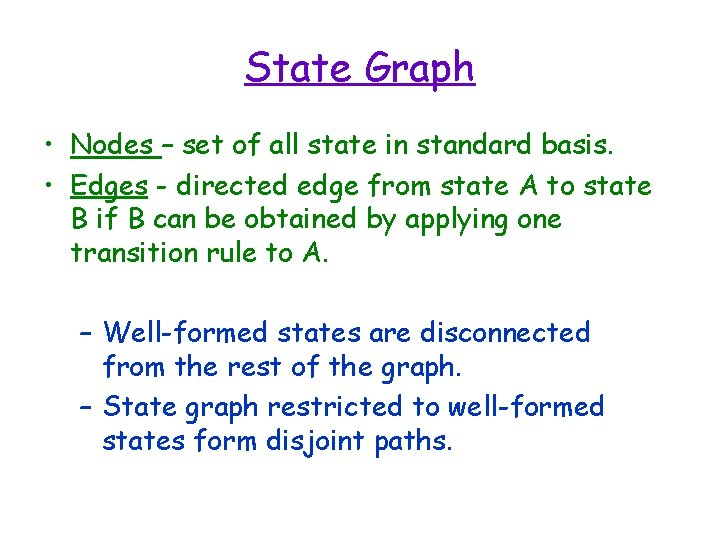State Graph • Nodes – set of all state in standard basis. • Edges - directed edge from state A to state B if B can be obtained by applying one transition rule to A. – Well-formed states are disconnected from the rest of the graph. – State graph restricted to well-formed states form disjoint paths.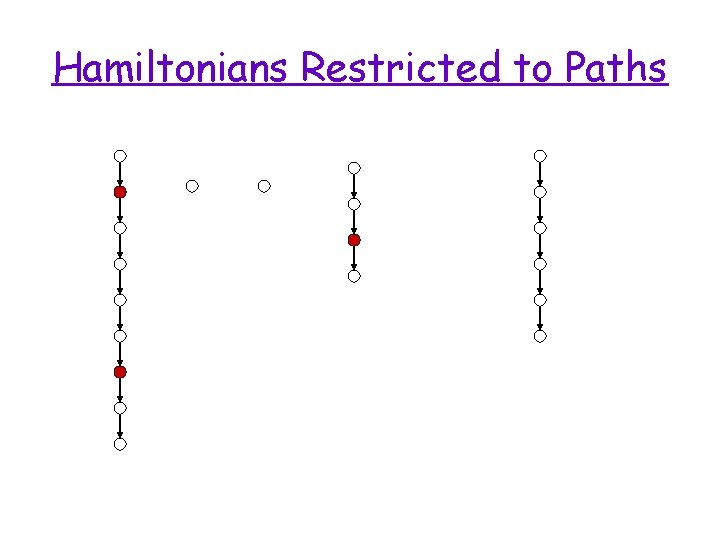Hamiltonians Restricted to PathsThe Hamiltonian restricted to a single path • If Hlegal has a non-zero entry, the minimum eigenvalue of H restricted to the subspace spanned by states in the path is W(1/l 3) which is W(1/n 6). [Kitaev 02] • If Hlegal is all zero, the state which is the uniform superposition of states in the path has zero energy.Bad States < e e < e u w < e e u w w g E > E E e e u u w g E < e 1 e u u w w w g E 0 > > • Will check for these states by showing that they evolve (via forward or backward application of the transition rules) to illegal states.How To Check for Bad States: An Example < e e u w w f E >How To Check for Bad States: An Example < e e e u u w w < e e u w w f w i > E >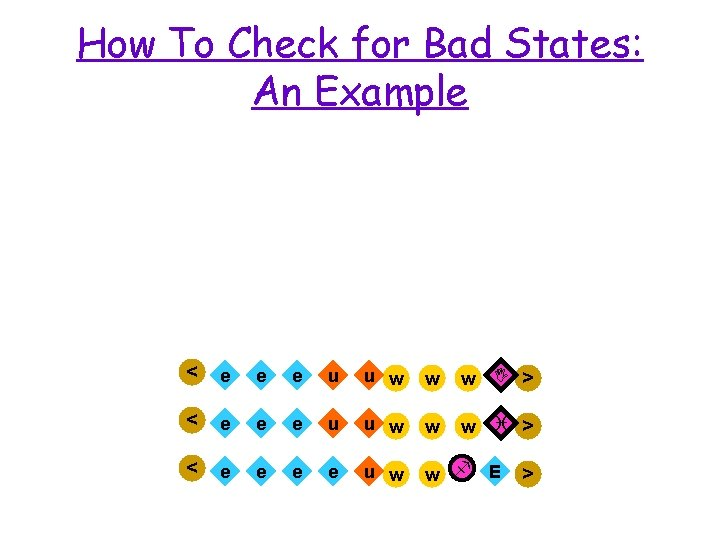How To Check for Bad States: An Example < e e e u u w w w I > < e e e u u w w w < e e u w w f i > E >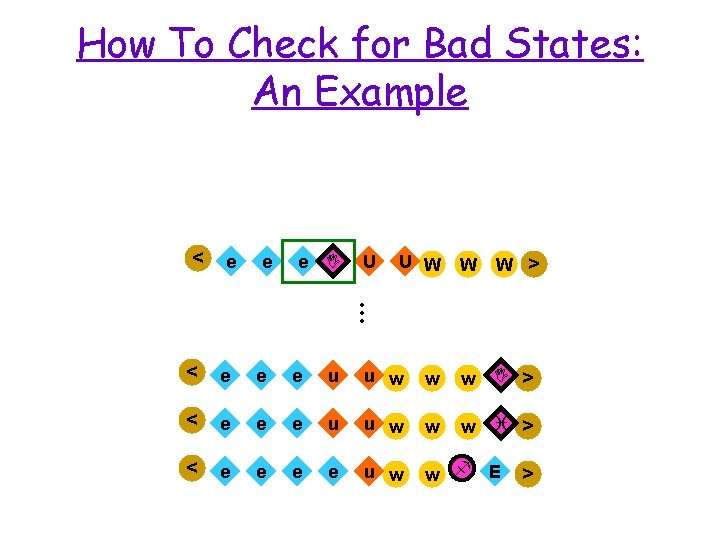How To Check for Bad States: An Example e I U U W W W > . . . < < e e e u u w w w I > < e e e u u w w w < e e u w w f i > E >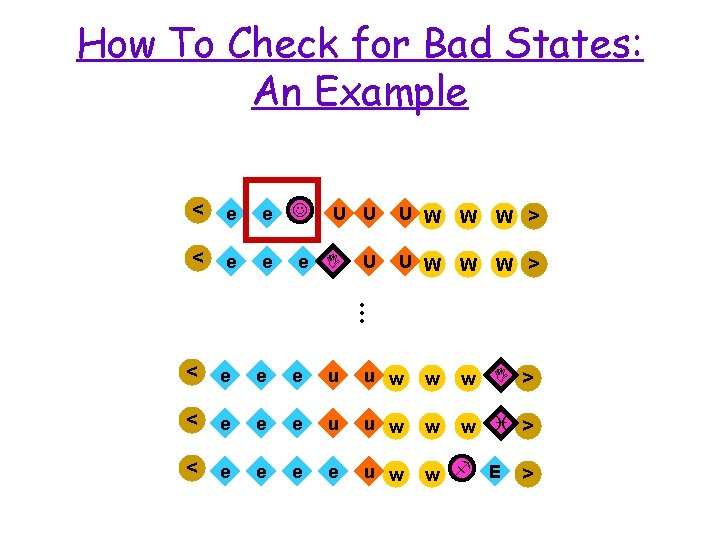How To Check for Bad States: An Example e e < e e J U U e I U U W W W > . . . < < e e e u u w w w I > < e e e u u w w w < e e u w w f i > E >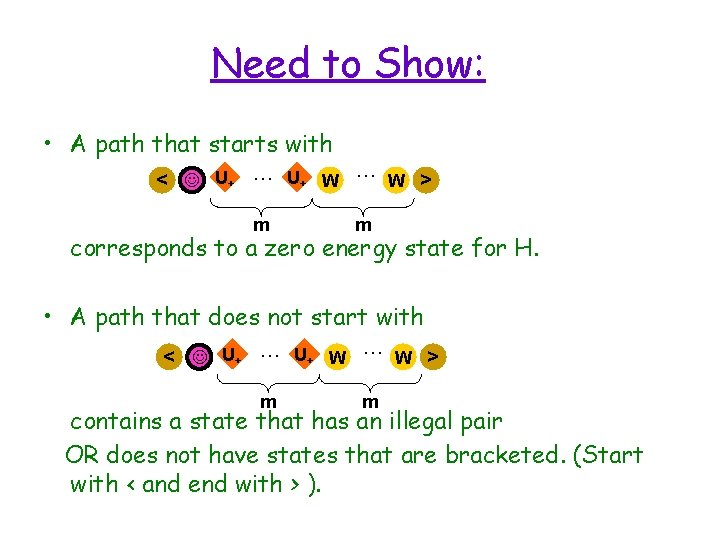Need to Show: • A path that starts with … < J U+ … U+ W m W > m corresponds to a zero energy state for H. • A path that does not start with …W > < J U+ … U+ W m m contains a state that has an illegal pair OR does not have states that are bracketed. (Start with < and end with > ).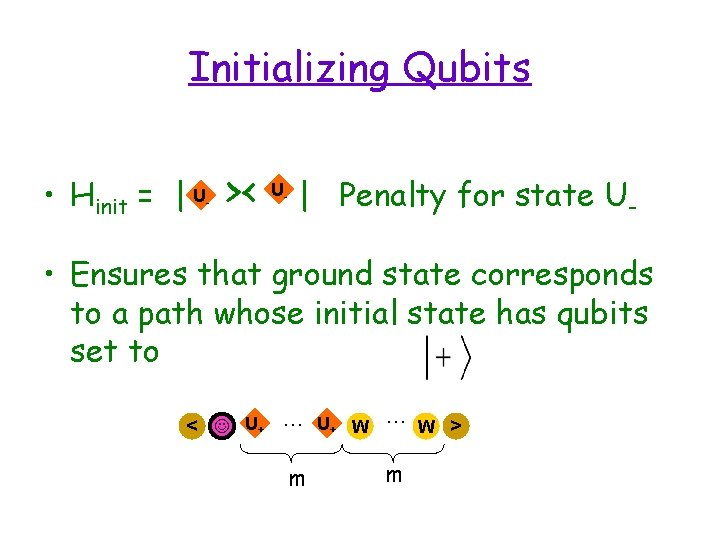Initializing Qubits • Hinit = | U - >< U - | Penalty for state U- • Ensures that ground state corresponds to a path whose initial state has qubits set to < J U+ … m U+ W … m W >Enforcing Bracketed States Hbracket = I - | < >< < | -| > >< > | H = 3( Htrans + Hlegal ) + Hinit + Hbracket – 3( Htrans + Hlegal ) term ensures there are no bracket terms in the middle. – Hbracket term gives an energy benefit for having brackets at the end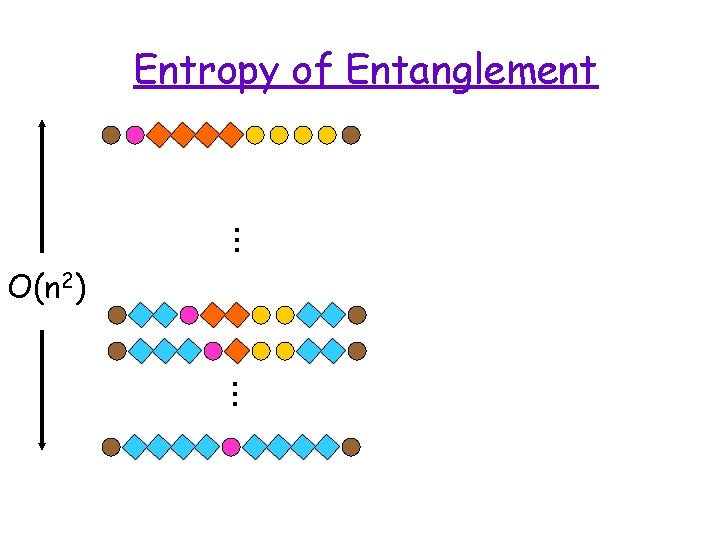… Entropy of Entanglement O(n 2) …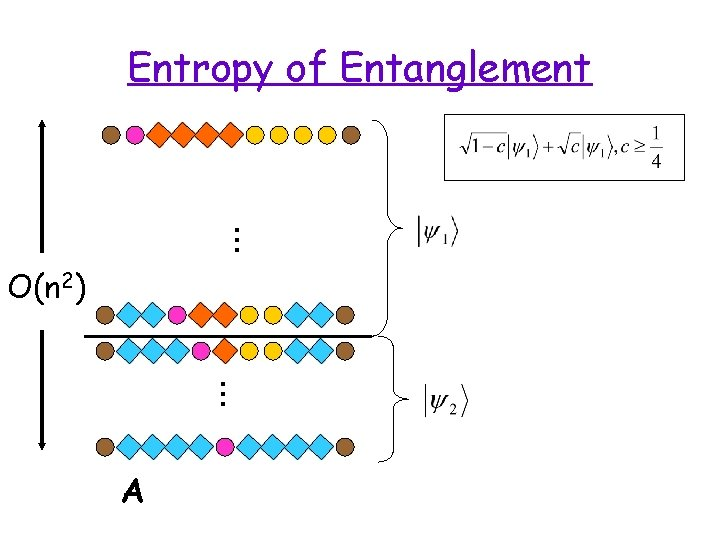… Entropy of Entanglement O(n 2) … A… Entropy of Entanglement O(n 2) … A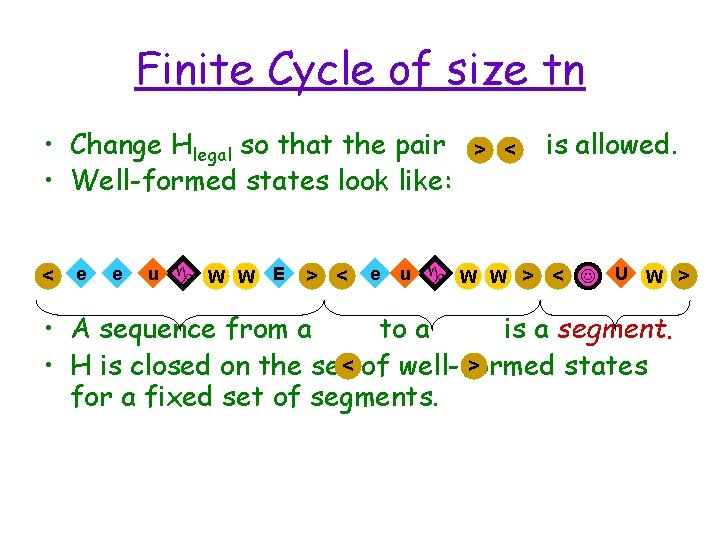Finite Cycle of size tn • Change Hlegal so that the pair • Well-formed states look like: < e e u g W W E > < e > < u g W W > is allowed. < J U W > • A sequence from a to a is a segment. > • H is closed on the set< of well-formed states for a fixed set of segments.Finite Cycle Cont. • H = p(n)(Hlegal + Htrans +Hinit) + Hsize • For p(n) large enough, using the Projection Lemma of Kempe-Kitaev-Regev, we can assume that the ground state of H is composed of tensor projects of ground states for finite chains. • Ground state for finite chain of length l is • Ground state for H will have form: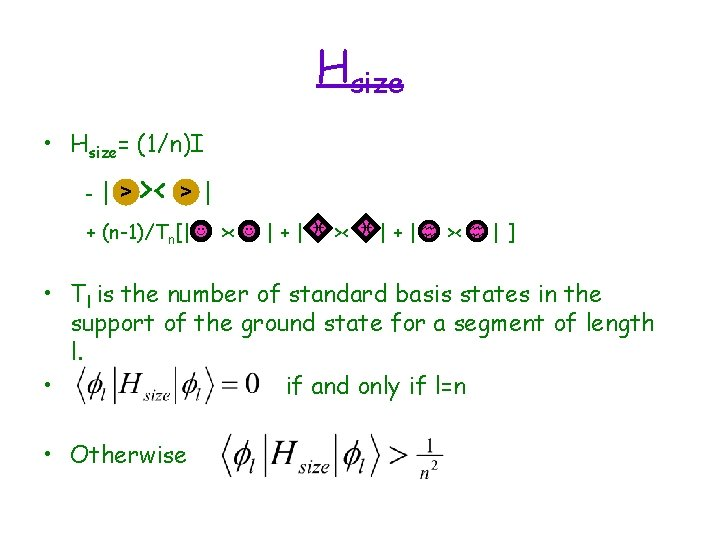Hsize • Hsize= (1/n)I - |> >< > | + (n-1)/Tn[| J >< J | + | i >< i | + | h >< h | ] • Tl is the number of standard basis states in the support of the ground state for a segment of length l. • if and only if l=n • Otherwise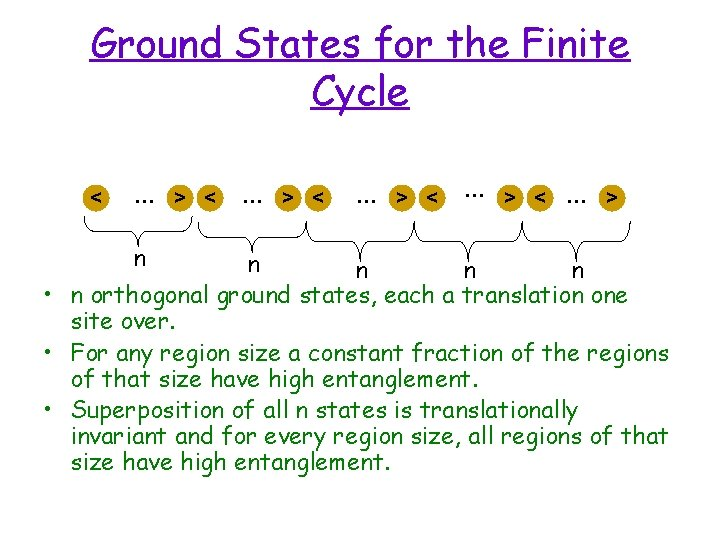Ground States for the Finite Cycle < … n > < … > n n • n orthogonal ground states, each a translation one site over. • For any region size a constant fraction of the regions of that size have high entanglement. • Superposition of all n states is translationally invariant and for every region size, all regions of that size have high entanglement.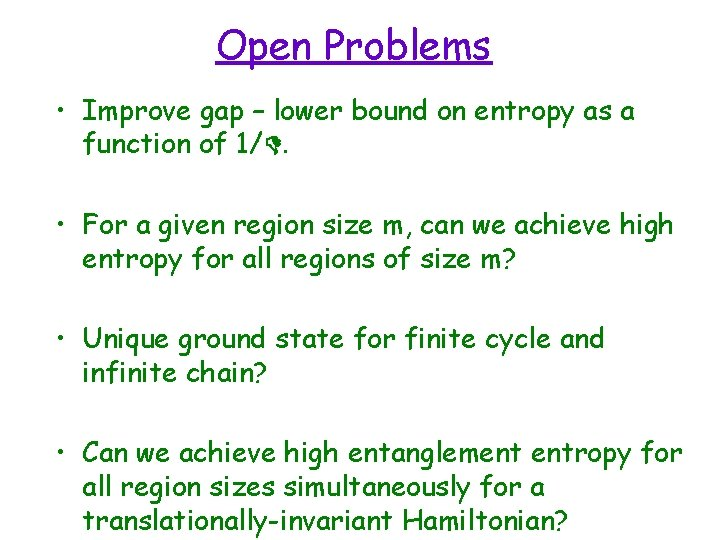Open Problems • Improve gap – lower bound on entropy as a function of 1/D. • For a given region size m, can we achieve high entropy for all regions of size m? • Unique ground state for finite cycle and infinite chain? • Can we achieve high entanglement entropy for all region sizes simultaneously for a translationally-invariant Hamiltonian?